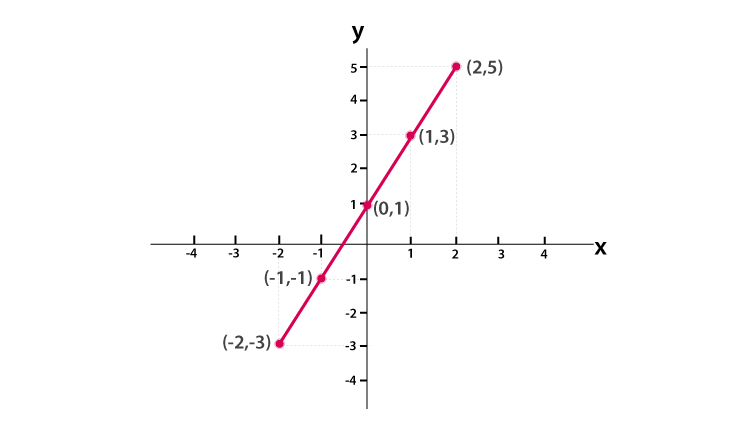# Linear Graph

Linear means straight and a graph is a diagram which shows a connection or relation between two or more quantity. So, the linear graph is nothing but a straight line or straight graph which is drawn on a plane connecting the points on x and y coordinates. We use linear relations in our everyday life, and by graphing those relations in a plane, we get a straight line.

Now that you have got an introduction to the linear graph let us explain it more through its definition and an example problem.

## Linear Graph Equation

As discussed, linear graph forms a straight line and denoted by an equation;

y=mx+c

where m is the gradient of the graph and c is the y-intercept of the graph.

The gradient between any two points (x1, y1) and (x2, y2) are any two points on the linear or straight line.

The value of gradient m is the ratio of the difference of y-coordinates to the difference of x-coordinates.

i.e. m=y2-y1/x2-xOr y-y1= m (x – x1)

The linear equation can also be written as,

ax + by + c = 0

where a,b and c are constants

### Linear Graph Examples

Let us understand the Linear graph definition with examples.

1. The equation y=2x+1 is a linear equation or forms a straight line on the graph. When the value of x increases, then ultimately the value of y also increases by twice of the value of x plus 1.
2. Suppose, if we have to plot a graph of a linear equation y=2x+1.

Let us consider y=2x+1 forms a straight line. Now, first, we need to find the coordinates of x and y by constructing the below table;

 x -2 -1 0 1 2 y

Now calculating value of y with respect to x, by using given linear equation,

y=2x+1

y=2(-2)+1= -3 for x=-2

y=2(-1)+1= -1 for x=-1

y=2(0)+1= 1 for x=0

y=2(1)+1= 3 for x=1

y=2(2)+1= 5 forx=2

So the table can be re-written as;

 x -2 -1 0 1 2 y -3 -1 1 3 5

Now based on these coordinates we can plot the graph as shown below.